## 10.5.2 Sequential Games on State Spaces

An apparent problem in the previous section is that the number of vertices grows exponentially in the number of stages. In some games, however, there may be multiple action sequences that lead to the same state. This is true of many popular games, such as chess, checkers, and tic-tac-toe. In this case, it is convenient to define a state space that captures the complete set of unique game configurations. The player actions then transform the state. If there are different action sequences that lead to the same state, then separate vertices are not needed. This converts the game tree into a game graph by declaring vertices that represent the same state to be equivalent. The game graph is similar in many ways to the transition graphs discussed in Section 10.1, in the sequential game against nature. The same idea can be applied when there are opposing players.

We will arrive at a sequential game that is played over a state space by collapsing the game tree into a game graph. We will also allow the more general case of costs occurring on any transition edges, as opposed to only the leaves of the original game tree. Only the stage-by-stage model from the game tree is generalized here. Generalizations that use other information models are considered in Section 11.7. In the formulation that follows,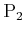can be can viewed as the replacement for nature in Formulation 10.1. The new formulation is still a generalization of Formulation 9.7, which was a single-stage, zero-sum game. To keep the concepts simpler, all spaces are assumed to be finite. The formulation is as follows.

Formulation 10..4 (Sequential Zero-Sum Game on a State Space)
1. Two players,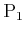and.
2. A finite, nonempty state space.
3. For each state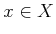, a finite, nonempty action space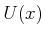for.
4. For each state, a finite, nonempty action space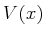for. To allow an extension of the alternating play model from Section 10.5.1,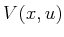could alternatively be defined, to enable the set of actions available toto depend on the action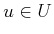of.
5. A state transition function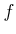that produces a state,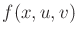, for every,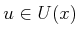, and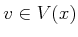.
6. A set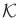of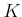stages, each denoted by, which begins at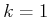and ends at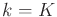. Let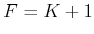, which is the final stage, after the last action is applied.
7. An initial state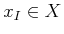. For some problems, this may not be specified, in which case a solution must be found from all initial states.
8. A stage-additive cost functional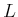. Let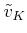denote the history of's actions up to stage. The cost functional may be applied to any combination of state and action histories to yield(10.106)

It will be assumed that both players always know the current state. Note that there are no termination actions in the formulation. The game terminates after each player has actedtimes. There is also no direct formulation of a goal set. Both termination actions and goal sets can be added to the formulation without difficulty, but this is not considered here. The action sets can easily be extended to allow a dependency on the stage, to yield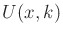and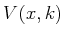. The methods presented in this section can be adapted without trouble. This is avoided, however, to make the notation simpler.

Subsections
Steven M LaValle 2020-08-14